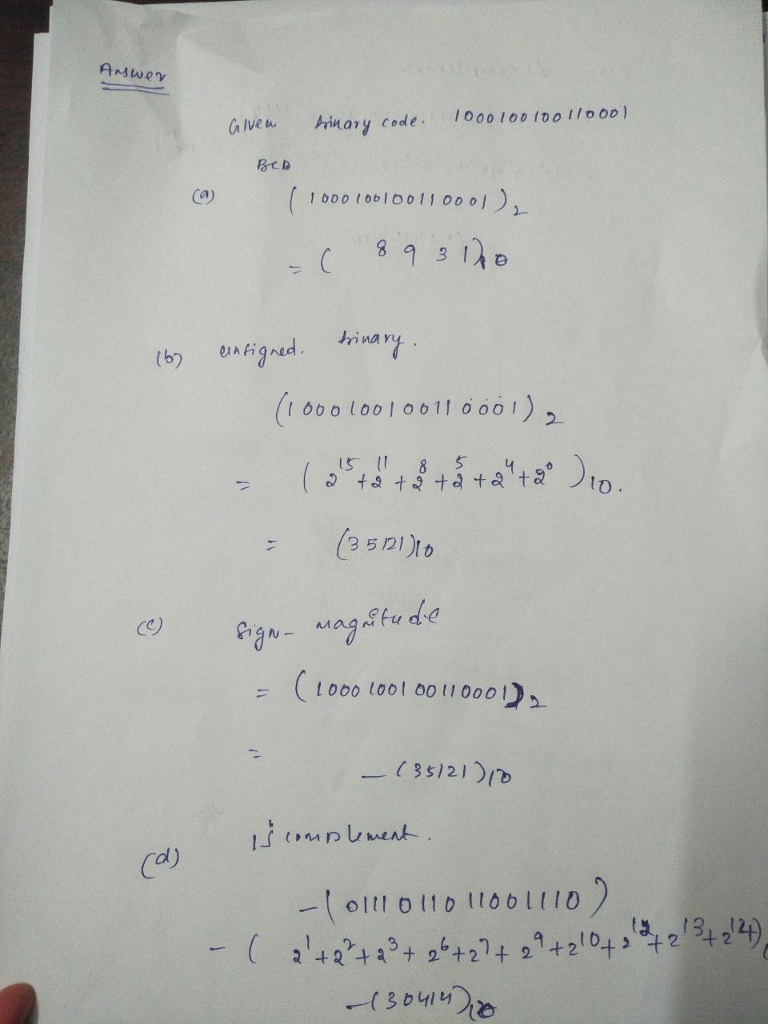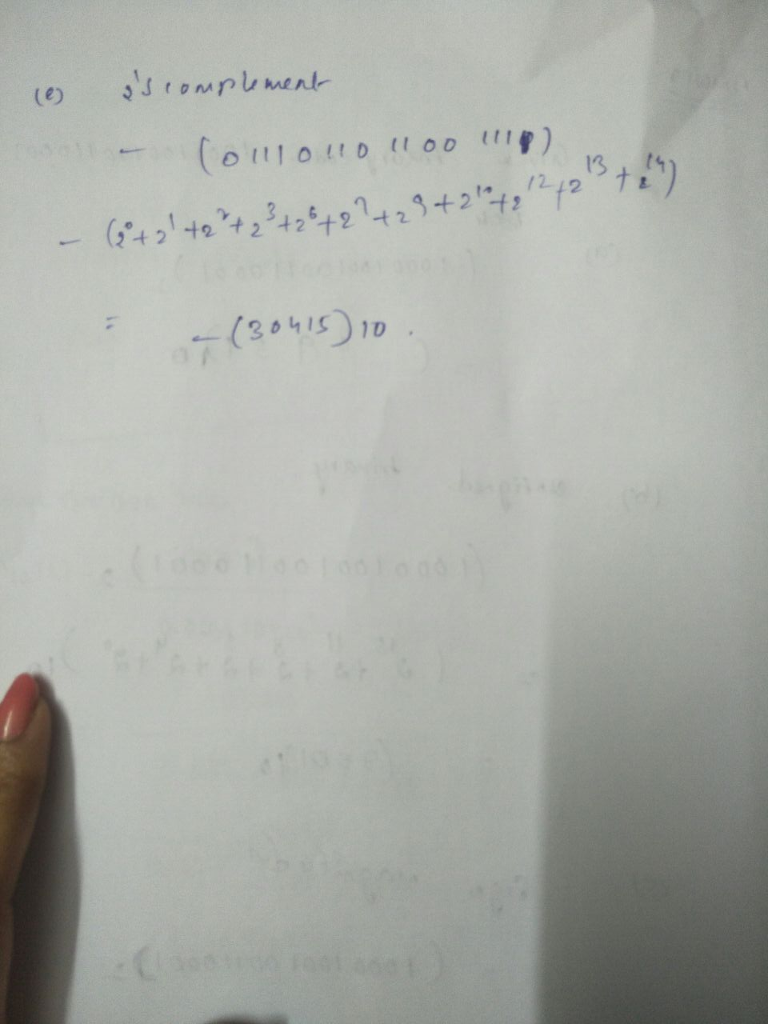# Homework Solution: Given the binary code 1000100100110001. Determine the meaning of this code if the code repr…

Given the binary code 1000100100110001. Determine the meaning of this code if the code represents (a) A BCD code (b) An unsigned integer (c) A signed integer in signed magnitude (d) A signed integer in 1’s complement (e) A signed integer in 2’s complement